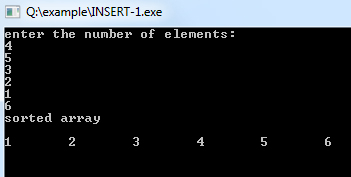## Data Structure Tutorial Index

Insertion sort is a to some extent an interesting algorithm with an expensive runtime characteristic having O(n2). This algorithm can be best thought of as a sorting scheme which can be compared to that of sorting a hand of playing cards, i.e., you take one card and then look at the rest with the intent of building up an ordered set of cards in your hand.

### Algorithm for Insertion Sort

1. algorithm Insertion_sort(list)
2. Pre: list 6= fi
3. Post: list has been sorted into values of ascending order
4. unsorted <- 1
5. while unsorted < list.Count
6. hold <- list[unsorted]
7. i Ã unsorted - 1
8. while i >= 0 and hold < list[i]
9. list[i + 1] <- list[i]
10.  i <- i - 1
11. end while
12. list[i + 1] <- hold
13. unsorted <- unsorted + 1
14. end while
15. return list
16. end Insertion_sort

Example:

``````#include <iostream>
#include <conio.h>

int main()
{
int a, i, j, k, tmp;
cout << "enter the number of elements\n";
for (i = 0; i < 6; i++) { cin >> a[i];
}
for (i = 1; i < 6; i++) { for (j = i; j >= 1; j--) {
if (a[j] < a[j - 1]) {
tmp = a[j];
a[j] = a[j - 1];
a[j - 1] = tmp;
}
else
break;
}
}
cout << "sorted array\n" << endl;
for (k = 0; k < 6; k++) {
cout << a[k] << "\t";
}
}``````

Output: